# First Law Applied to Flow Processes Notes | Study Mechanical Engineering SSC JE (Technical) - Mechanical Engineering

## Mechanical Engineering: First Law Applied to Flow Processes Notes | Study Mechanical Engineering SSC JE (Technical) - Mechanical Engineering

The document First Law Applied to Flow Processes Notes | Study Mechanical Engineering SSC JE (Technical) - Mechanical Engineering is a part of the Mechanical Engineering Course Mechanical Engineering SSC JE (Technical).
All you need of Mechanical Engineering at this link: Mechanical Engineering

FIRST LAW APPLIED TO FLOW PROCESSES

In a flow if fluid properties do not change with time at any given location, the process is steady flow process.

•  In steady flow process there is no accumulation of mass or energy in control volume i.e. conservation of mass and energy occurs.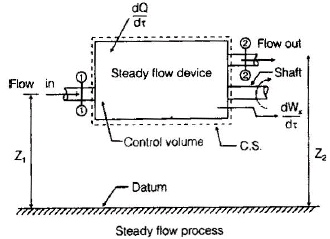A1, A2 — cross-section of stream, (m2)
m1, m2 — mass flow rate, (kg/s)
p1, p2 — pressure, (absolute), (N/m2)
v1, v— specific volume, (m3/kg)
u1, u2 — specific internal energy, (J/kg)
V1, V2 — velocity, (m/s)
Z1, Z2 — elevation above an arbitrary datum, (m)

dQ/dt— net rate of heat transfer through the control surface, (J/s)

dWx/dt—net rate of work transfer through the control surface, (J/s)
t – time(s)

Now,
By conservation of mass

m1 = m2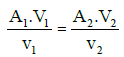m1, m2 — mass flow rate entering and leaving the control volume.

•  Conservation of Energy (Steady flow energy equation SFEE)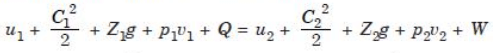u= internal energy per kg of fluid

C= velocity of fluid

• Per unit mass basis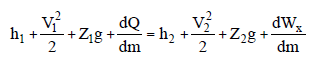a)For one fluid stream SFEE (per unit time) is used.

b)For more than one fluid stream SFEE (per unit mass) is used.

c)Application of SFEE is steady flow processes

Nozzless & Diffusers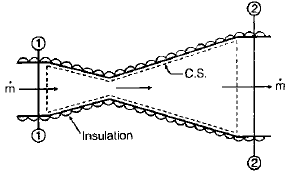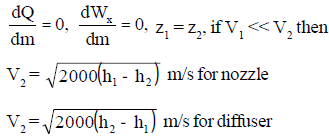where, h is in kJ/ kg

•  A nozzle is a device which increases the velocity or K.E. of fluid at the expense of its pressure drop.
•  A diffuser is a device which increases the pressure of fluid at the expense of K.E.

Throttling Device

Throttling device is the generic name of any device or process that simply dissipates pressure energy m˙pv by irreversibly converting it into thermal energy. Unlike nozzles and diffusers, throttling devices provide no form of useful energy recovery.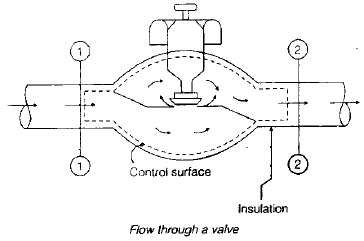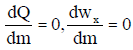z1 = z2, V1, V2 are negligible
h1 = h2

•  When a fluid flow through a narrow passage like an orifice, partially opened valve, there is an appreciable drop in pressure. The process is throttling process.
•  Throttling is an isenthalpic process.

Turbine and Compressor

Turbine is the device in which fluid expands. During the expansion work will be done by the fluid to drive, for example, electric generation. In this case, power output occurs.

Compressor is the device which is used to compress the fluid and increase its pressure. That means power input is required.

• The 1st law of thermodynamics: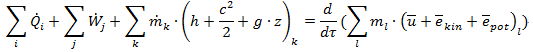The change of kinetic energy and potential energy of fluid flowing into and out of turbines and compressors are very small that can usually be neglected:

(ekin)out – (ekin)in≈0 → c2out – c2in≈0

(epot)out – (epot)in≈0 → g•(zout – zin)≈0

Turbine and compressors are also regarded as steady-flow engineering device, so the term at the right-hand side equals zero: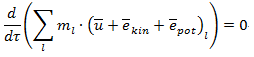Furthermore, mout=min because of conservation of mass.

So now we obtain a simplified expression for turbine and compressor:

q+w+ hin – hout=0

where:

• q=heat transferred per unit mass
• w=work done by turbine or on compressor per unit mass
• hin= specific enthalpy of inlet fluid
• hout= specific enthalpy of outlet fluid

As discussed above,

• w<0 → turbine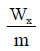= (h1 - h2) for turbine

• w>0 → compressor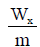= (h2 - h1) for compressor

Heat Exchanger

• A heat exchanger is a system used to transfer heat between two or more fluids. Heat exchangers are used in both cooling and heating processes. The fluids may be separated by a solid wall to prevent mixing or they may be in direct contact.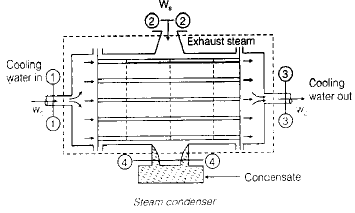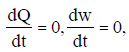z1 = z2, V1, V2 are negligible

The document First Law Applied to Flow Processes Notes | Study Mechanical Engineering SSC JE (Technical) - Mechanical Engineering is a part of the Mechanical Engineering Course Mechanical Engineering SSC JE (Technical).
All you need of Mechanical Engineering at this link: Mechanical EngineeringUse Code STAYHOME200 and get INR 200 additional OFF

## Mechanical Engineering SSC JE (Technical)

6 videos|89 docs|55 tests

Track your progress, build streaks, highlight & save important lessons and more!

,

,

,

,

,

,

,

,

,

,

,

,

,

,

,

,

,

,

,

,

,

;